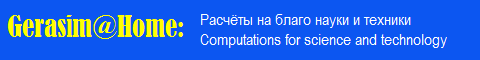Российские распределенные вычисления на платформе BOINCBerkeley Open Infrastructure for Network Computing

 Welcome Guest

Project News

 Monday, October 19, 2020 evatutin #96

Each partial loop is a loop in a diagonal Latin square by definition. Similarly, by definition, each intercalate is a partial loop of length 4. These simple statements allow us to establish a number of relationships between the values of the numerical series associated with loops and intercalates and calculated earlier:

1. For loops: 0 <= A307166(n) <= A307167(n).
2. For partial loops: 0 <= A307170(n) <= A307171(n).
3. For minimum values: 0 <= A307163(n) <= A307170(n) <= A307166(n).
4. For maximum values: A307164(n) <= A307171(n) <= A307167(n).

Add to OEIS, the first swallow (https://oeis.org/draft/A307163) went, or rather, flew :)